The OPTQP Procedure

Example 12.3 Portfolio Selection with Transactions

Consider a portfolio selection problem with a slight modification. You are now required to take into account the current position and transaction costs associated with buying and selling assets. The objective is to find the minimum variance portfolio. In order to understand the scenario better, consider the following data.

You are given three assets. The current holding of the three assets is denoted by the vector= [200, 300, 500], the amount of asset bought and sold is denoted by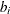and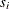, respectively, and the net investment in each asset is denoted byand is defined by the following relation: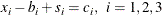Suppose you pay a transaction fee of 0.01 every time you buy or sell. Let the covariance matrix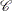be defined as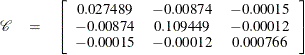Assume that you hope to obtain at least 12% growth. Let= [1.109048, 1.169048, 1.074286] be the vector of expected return on the three assets, and let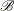=1000 be the available funds. Mathematically, this problem can be written in the following manner: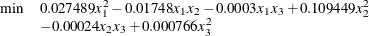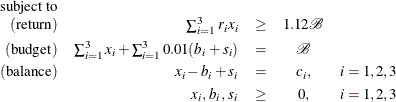The QPS-format input data set is as follows:

data potrdata;
input field1 $field2$ field3 $field4 field5$ field6 @;
datalines;
NAME    .         POTRAN    .            .          .
ROWS    .         .         .            .          .
N       OBJ.FUNC  .         .            .          .
G       RETURN    .         .            .          .
E       BUDGET    .         .            .          .
E       BALANC1   .         .            .          .
E       BALANC2   .         .            .          .
E       BALANC3   .         .            .          .
COLUMNS .         .         .            .          .
.       X1        RETURN    1.109048     BUDGET     1.0
.       X1        BALANC1   1.0          .          .
.       X2        RETURN    1.169048     BUDGET     1.0
.       X2        BALANC2   1.0          .          .
.       X3        RETURN    1.074286     BUDGET     1.0
.       X3        BALANC3   1.0          .          .
.       B1        BUDGET    .01          BALANC1   -1.0
.       B2        BUDGET    .01          BALANC2   -1.0
.       B3        BUDGET    .01          BALANC3   -1.0
.       S1        BUDGET    .01          BALANC1    1.0
.       S2        BUDGET    .01          BALANC2    1.0
.       S3        BUDGET    .01          BALANC3    1.0
RHS     .         .         .            .          .
.       RHS       RETURN    1120         .          .
.       RHS       BUDGET    1000         .          .
.       RHS       BALANC1   200          .          .
.       RHS       BALANC2   300          .          .
.       RHS       BALANC3   500          .          .
RANGES  .         .         .            .          .
BOUNDS  .         .         .            .          .
QUADOBJ .         .         .            .          .
.       X1        X1        0.054978     .          .
.       X1        X2        -.01748      .          .
.       X1        X3        -.0003       .          .
.       X2        X2        0.218898     .          .
.       X2        X3        -.00024      .          .
.       X3        X3        0.001532     .          .
ENDATA  .         .         .            .          .
;

Use the following SAS statements to solve the problem:

proc optqp data=potrdata
primalout = potrpout
printlevel = 0
dualout   = potrdout;
run;

The optimal solution is displayed in Output 12.3.1.

Output 12.3.1: Portfolio Selection with Transactions

 The OPTQP Procedure Primal Solution

Obs Objective Function ID RHS ID Variable
Name
Variable
Type
Linear
Objective
Coefficient
Lower
Bound
Upper Bound Variable Value Variable
Status
1 OBJ.FUNC RHS X1 N 0 0 1.7977E308 397.584 O
2 OBJ.FUNC RHS X2 N 0 0 1.7977E308 406.115 O
3 OBJ.FUNC RHS X3 N 0 0 1.7977E308 190.165 O
4 OBJ.FUNC RHS B1 N 0 0 1.7977E308 197.584 O
5 OBJ.FUNC RHS B2 N 0 0 1.7977E308 106.115 O
6 OBJ.FUNC RHS B3 N 0 0 1.7977E308 0.000 O
7 OBJ.FUNC RHS S1 N 0 0 1.7977E308 0.000 O
8 OBJ.FUNC RHS S2 N 0 0 1.7977E308 0.000 O
9 OBJ.FUNC RHS S3 N 0 0 1.7977E308 309.835 O